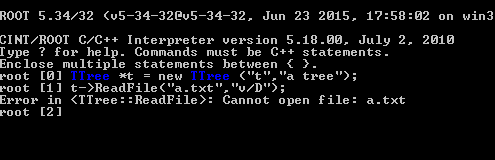# Read txt file and draw histogram

Ok I deleted other a.txt and I downloaded a.txt which your attachement and I try write this code
Shoul I ignored it and I go on wirte this code or Is it a problem? What should I do?The “a.txt” file must be in the same subdirectory in which you run “root” (i.e. where your old broken file, which you deleted, was), or you must specify the “full path” to it in the “ReadFile” call.

YESS Thank you so much problem is solved But Can I ask questions I want to find that my result is proportional each other What I mean is that my result can create a pol0 I go to fit panel and selected pol0 but I havent got a result

Click the “Fit” button (note: in the “Fit Options”, you should probably “Use range” when fitting, removing the very first and the very last bins -> zoom the histogram with a mouse from -3 to 3, or use the “X” slider down in the “Fit Panel”).

Thank you so much It’s ok

I have" data.txt "file in the form of three columns : x,y,and z
I tried a method to draw it and then convert it to a root file for easy handling but it does not give me a file and does not show results
I want to draw this data ,
x y z
|13|1000|42|
|13|10000|450|
|13|1000000|43369|
|29|1000|8|
|29|10000|80|
|29|1000000|9004|
|4|1000|70|
|4|10000|714|
|4|1000000|71721|
|79|1000|1|
|79|10000|16|
|79|1000000|2172|

method1:
#include “TRandom.h”
#include “TFile.h”
#include “TNtuple.h”

void test(){

TNtuple data (“ntuple”, “data.txt”,“x:y:z”);

for (int i =0;i<10000;++i){

float x = gRandom->Uniform(4,79);
float y = gRandom->Uniform(1000,1000000);
float z = gRandom->Uniform(1,71721);

data.Fill(x,y,z);
}
TFile f(“ntuple.root”,“RECREATE”);
data.write();
f.close();
}

method2:
#include “TRandom.h”
#include “TFile.h”
#include “TTree.h”

void ExampleTree(const char*filename=“tree.root”);
{
TTree data(“tree”,“data.txt”);
double x,y,z;

data.Branch(“x”.&x,“x/D”);
data.Branch(“y”.&x,“y/D”);
data.Branch(“z”.&z,“z/D”);

for (int i =0;i<10000;++i){

float x = gRandom->Uniform(4,79);
float y = gRandom->Uniform(1000,1000000);
float z = gRandom->Uniform(1,71721);

data.Fill();
}
TFile f(filename,“RECREATE”);
data.write();
f.close();
}

Both methods did not get a file root and did not draw a drawing ??

#include “Riostream.h”
void tree() {
ifstream in;
in.open(Form(“data.txt”));
Float_t x,y,z;
Int_t nlines = 0;
TFile *f = new TFile(“data.root”,“RECREATE”);
//TH1F *h1 = new TH1F(“h1”,“x distribution”,100,-4,4);
//TH2F *h12 = new TH2F(“h12”,“x:y distribution”,100,4,79,100,1000,1000000);
TH3F *h123 = new TH3F(“h123”,“x:y:z distribution”,5,1000,1000000,5,8,71721,5,4,79);
TNtuple *ntuple = new TNtuple(“ntuple”,“data fromascii file”,“x:y:z”);

while (1) {
in >> x >> y >> z;
if (!in.good()) break;
if (nlines =36 ) printf(“x=%f, y=%f, z=%f\n”,x,y,z);
//h1->Fill(x);
//h12->Fill(x,y);
h123->Fill(x,y,z);
ntuple->Fill(x,y,z);
nlines++;
}
printf(" found %d points\n",nlines);
in.close();
f->Write();
f->Close();
}

this is gooood

1 Like